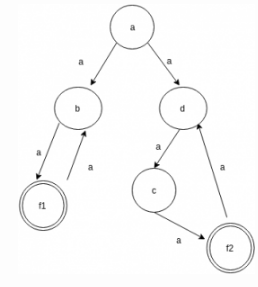Courses

# Test: Mealy Machine

## 10 Questions MCQ Test Theory of Computation | Test: Mealy Machine

Description
This mock test of Test: Mealy Machine for Computer Science Engineering (CSE) helps you for every Computer Science Engineering (CSE) entrance exam. This contains 10 Multiple Choice Questions for Computer Science Engineering (CSE) Test: Mealy Machine (mcq) to study with solutions a complete question bank. The solved questions answers in this Test: Mealy Machine quiz give you a good mix of easy questions and tough questions. Computer Science Engineering (CSE) students definitely take this Test: Mealy Machine exercise for a better result in the exam. You can find other Test: Mealy Machine extra questions, long questions & short questions for Computer Science Engineering (CSE) on EduRev as well by searching above.
QUESTION: 1

### Which of the following does not belong to input alphabet if S={a, b}* for any language?

Solution:

The automaton may be allowed to change its state without reading the input symbol using epsilon but this does not mean that epsilon has become an input symbol. On the contrary, one assumes that the symbol epsilon does not belong to any alphabet.

QUESTION: 2

### The number of final states we need as per the given language?Language L: {an| n is e ven or divisible by 3}

Solution: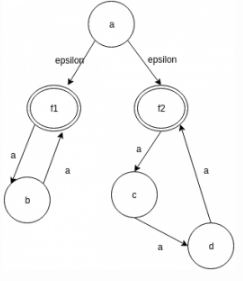QUESTION: 3

### An e-NFA is ___________ in representation.

Solution:

An e-NFA consist of 5 tuples: A=(Q, S, d, q0. F)
Note: e is never a member of S.

QUESTION: 4

State true or false:Statement: Both NFA and e-NFA recognize exactly the same languages.

Solution:

e-NFA do come up with a convenient feature but nothing new.They do not extend the class of languages that can be represented.

QUESTION: 5

Design a NFA for the language:
L: {an| n is even or divisible by 3}
Which of the following methods can be used to simulate the same.

Solution:
QUESTION: 6

Which of the following belongs to the epsilon closure set of a?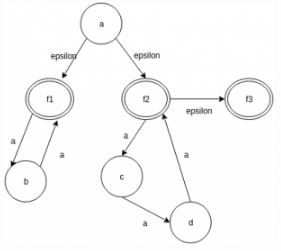Solution:

The epsilon closure of the set q is the set that contains q, together with all the states which can be reached starting at q by following only epsilon transitions.

QUESTION: 7

The number of elements present in the e-closure(f2) in the given diagram: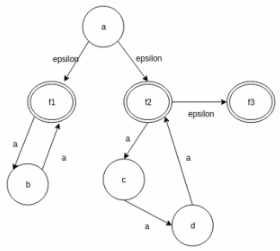Solution:

The epsilon closure set of f2 consist of the elements:{f2, f3}. Thus the count of the element in the closure set is 2.

QUESTION: 8

Which of the steps are non useful while eliminating the e-transitions for the given diagram?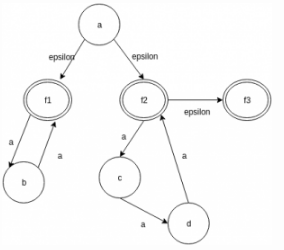Solution:

The given are the steps followed while eliminating epsilon transitions from a NFA or converting an e-NFA to just NFA.

QUESTION: 9

Is the language preserved in all the steps while eliminating epsilon transitions from a NFA?

Solution:

Yes, the language is preserved during the dteps of construction: L(N)=L(N1)=L(N2)=L(3).

QUESTION: 10

Remove all the epsilon transitions in the given diagram and compute the number of a-transitions in the result?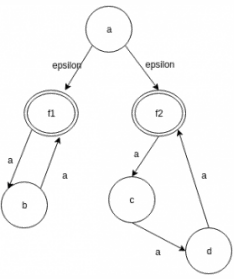Solution: